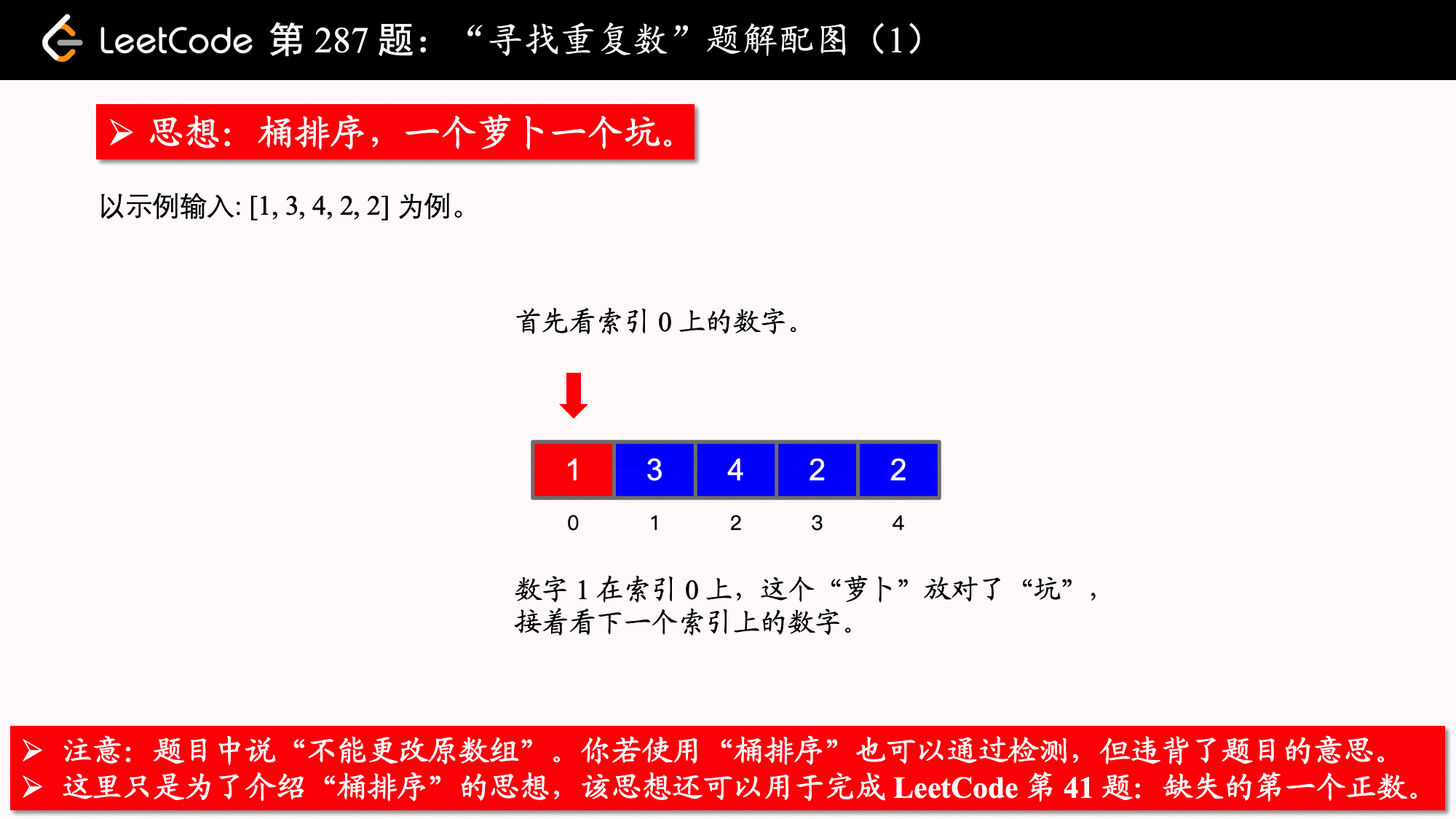Languages: Python
Categories: 算法分析
Solution.java
public class Solution {

public int findDuplicate(int[] nums) {
int len = nums.length;
for (int i = 0; i < len; i++) {
while (nums[i] != i + 1) {
if (nums[i] == nums[nums[i] - 1]) {
return nums[i];
}
swap(nums, i, nums[i] - 1);
}
}
// 数组中没有重复的整数，测试用例错误
return 0;
}

private void swap(int[] nums, int index1, int index2) {
if (index1 == index2) {
return;
}
int temp = nums[index1];
nums[index1] = nums[index2];
nums[index2] = temp;
}
}

0287-寻找重复数（桶排序）.py
from typing import List

class Solution:
def findDuplicate(self, nums: List[int]) -> int:
# 桶排序，数字 i 应该在索引 i - 1 上，否则交换
size = len(nums)
for i in range(size):
while nums[i] != i + 1:
if nums[i] == nums[nums[i] - 1]:
return nums[i]
self.__swap(nums, i, nums[i] - 1)

def __swap(self, nums, index1, index2):
if index1 == index2:
return
nums[index1], nums[index2] = nums[index2], nums[index1]


### Comment list( 1 )2019-07-12 09:281、nums[i] != i + 1：想一想正确放置的情况，nums = 1nums = 2，依次类推，这一步是在判断，在遍历的时候，当前索引上的放置的元素的值是不是正确的“萝卜”；

2、if nums[i] == nums[nums[i] - 1]:：如果不是正确的“萝卜”，就得根据当前索引上的数值，看一看这个数字应该放在哪个位置上。例如数字 4 应该放在索引 3 上，那么就要检查数字 4（这里是 nums[i]），与索引 3 （这里是 nums[i] - 1）上的数值，即 nums[nums[i] - 1] 是否相等，如果相等，返回这个重复数，如果不相等，交换。

3、交换我单独写了一个方法，否则中括号会把自己绕晕。

• 时间复杂度：$O(N^2)$，这里需遍历一次整个数组，在遍历的时候还有一个 for 循环，因此时间复杂度为 $O(N^2)$。
• 空间复杂度：$O(1)$，这里无需使用额外的辅助空间，因此空间复杂度为 $O(1)$。